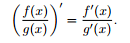# Freshman Dream Quotient Rule

• A
JasMath33
I have been interested in this idea of the FDQR. This idea states the following.I have been trying to see if there is some 2 functions which make this true, but have not found it on research or with trying functions. Does anyone have any insight on this. I think it is just neat and want to know more about what people know about it.

## Answers and Replies

DuckAmuck
What ever satisifies:

$$g' = g^2$$

and

$$f' = f \frac{g^2}{g-1}$$

Staff Emeritus
Homework Helper
There are infinitely many solutions. Given any function ##g##, the associated function can be found by solving the following ODE:

$$f' g (g - g') = (g')^2 f$$

So for example, let ##g(x) = e^x##. Then this reduces to ##f(x) = 0##.
If ##g(x) = x^2##, then
$$f'(x) x^2 (x^2 - 2x) = 4x^2 f(x)$$
or
$$\frac{f'(x)}{f(x)} = \frac{4}{x(x-2)}$$
Integrating yields
$$\log(f(x)) = 2\log(2-x) - 2\log(x)$$
or
$$f(x) = \frac{(2-x)^2}{x^2}$$
In general, given any function ##g## not satisfying ##g=g'## or ##g=0##, we can find an associated function ##f## by
$$f(x) = g(x) + e^{\int \frac{g'}{g-g'}}$$

•DuckAmuck
Gold Member
##f′g(g−g′)=(g′)^2f##

I think there a minus ##f'g(g-g')=-g'^{2}f## ...

•SammyS